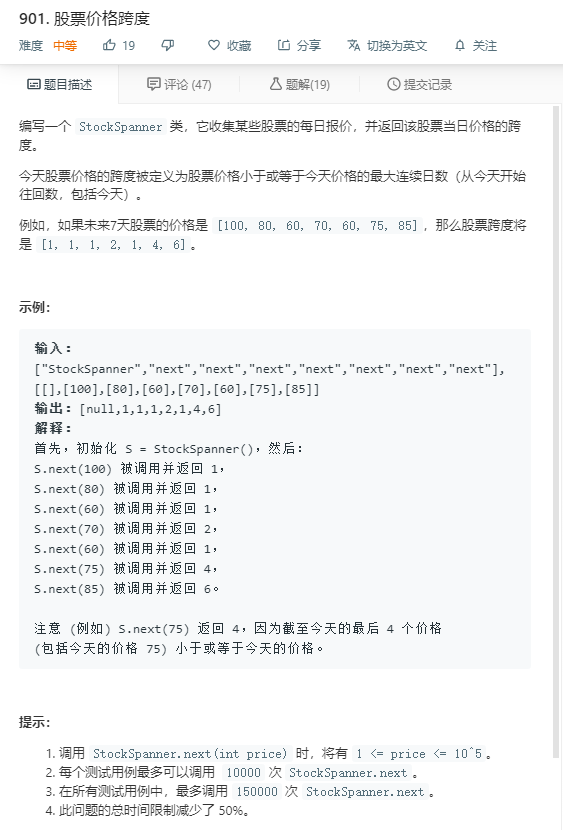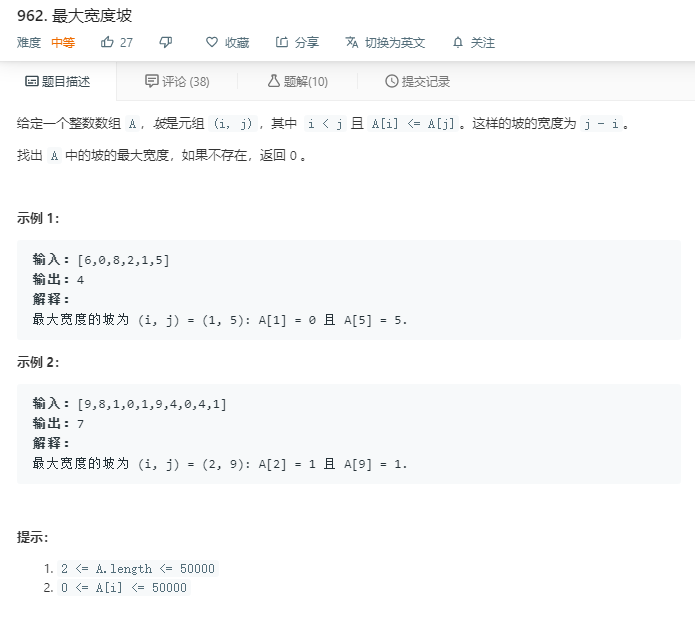# 单调栈的应用

## 应用

• 最基础的应用就是给定一组数，针对每个数，寻找它和它右边第一个比它大的数之间有多少个数。

• 给定一序列，寻找某一子序列，使得子序列中的最小值乘以子序列的长度最大。

• 给定一序列，寻找某一子序列，使得子序列中的最小值乘以子序列所有元素和最大。

### 901. 股票价格跨度#### 我的解法

class StockSpanner:
def __init__(self):
self.stack = []

def next(self, price: int) -> int:
degree = 1
while self.stack and self.stack[-1]<=price:
degree += self.stack.pop()
self.stack.append([price,degree])
return degree



#### Note:

• 找股票价格小于等于当前价格的最大连续日数等价于 找 从当前日子往前数第一个比当前股价大的日子的天数差
• 从而可以用单调栈解决问题 --> 可以获取左边第一个或者右边第一个比当前位大或者小的数。

### 962. 最大宽度坡#### 我的解法

class Solution:
def maxWidthRamp(self, A: List[int]) -> int:
stack = []
maxiter = 0
stack.append(0)
for i in range(1,len(A)):
if(A[i]<=A[stack[-1]]):
stack.append(i)
    for i in range(len(A)-1,-1,-1):
while(stack and A[i]>=A[stack[-1]]):
maxiter = max(maxiter,i-stack.pop())
return maxiter



• 我真的太菜啦。